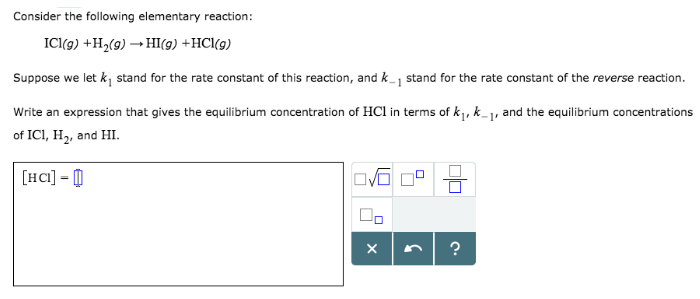Chemistry Practice Problems Equilibrium Expressions Practice Problems Solution: Consider the following elementary reaction: ICl (g...

🤓 Based on our data, we think this question is relevant for Professor Raineri's class at SUNY.

# Solution: Consider the following elementary reaction: ICl (g) + H2 (g) → HI (g) + HCl (g) Suppose we let k1 stand for the rate constant of this reaction, and k-1 stand for the rate constant of the reverse reaction. Write an expression that gives the equilibrium concentration of HCl in terms of k1, k-1, and the equilibrium concentrations of ICl, H2, and HI.

###### Problem

Consider the following elementary reaction:

ICl (g) + H2 (g) → HI (g) + HCl (g)

Suppose we let k1 stand for the rate constant of this reaction, and k-1 stand for the rate constant of the reverse reaction. Write an expression that gives the equilibrium concentration of HCl in terms of k1, k-1, and the equilibrium concentrations of ICl, H2, and HI.View Complete Written Solution

Equilibrium Expressions

Equilibrium Expressions

#### Q. Phosgene (carbonyl chloride), COCl2, is an extremely toxic gas that is used in manufacturing certain dyes and plastics. Phosgene can be produced by re...

Solved • Mon Sep 17 2018 18:51:01 GMT-0400 (EDT)

Equilibrium Expressions

#### Q. For the reaction shown here 2NH3 (g) ⇌ 3 H2 (g) + N2 (g) the equilibrium concentrations were found to be [NH3] = 0.250 M [H2] = 0.540 M [N2] = 0.800 M...

Solved • Fri Sep 07 2018 12:21:22 GMT-0400 (EDT)

Equilibrium Expressions

#### Q. Calculate the value of Kp for the equation C (s) + CO2 (g) ⇌ 2CO (g)                       Kp = ? given that at a certain temperature C (s) + 2H2O (g)...

Solved • Thu Sep 06 2018 17:20:27 GMT-0400 (EDT)

Equilibrium Expressions

#### Q. Write the following equilibrium-constant expressions: (a) Kc for Cr (s) + 3Ag+ (aq) ⇌ Cr3+ (aq) + 3Ag (s), (b) Kp for 3Fe (s) + 4H2O(g) ⇌ Fe3O4 (s) + ...

Solved • Wed Sep 05 2018 17:19:58 GMT-0400 (EDT)